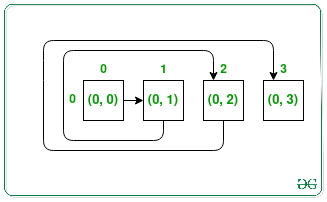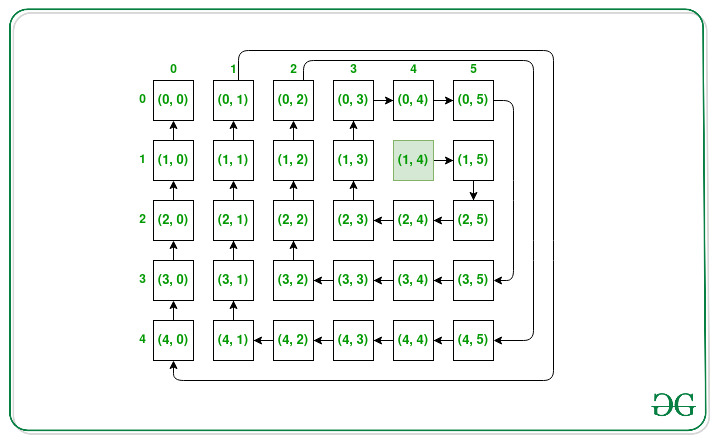Open in App
Not now

# Matrix Spiral Traversal starting from given Coordinates

• Difficulty Level : Expert
• Last Updated : 31 Mar, 2022

Given the order of the matrix N and M, and a source location (X, Y), the task is to find all the coordinates of the matrix in order when visited in clockwise spiral order((i.e. East-> South-> West-> North). Whenever moved outside the matrix, continue the walk outside it to return to the matrix later.

Examples:

Input: N = 1, M = 4, X = 0, Y = 0
Output: {{0, 0}, {0, 1}, {0, 2}, {0, 3}}
Explanation:Input: N = 5, M = 6, X = 1, Y = 4
Output: {{1, 4}, {1, 5}, {2, 5}, {2, 4}, {2, 3}, {1, 3}, {0, 3}, {0, 4}, {0, 5}, {3, 5}, {3, 4}, {3, 3}, {3, 2}, {2, 2}, {1, 2}, {0, 2}, {4, 5}, {4, 4}, {4, 3}, {4, 2}, {4, 1}, {3, 1}, {2, 1}, {1, 1}, {0, 1}, {4, 0}, {3, 0}, {2, 0}, {1, 0}, {0, 0}}
Explanation:Approach: The given problem can be solved by traversing the matrix from the position (X, Y) in a clockwise spiral direction, and whenever reached outside the matrix, don’t include the coordinates in the solution but continue traversing outside to reach again inside the matrix. Continue this process until all cells of the matrix are not included in the solution or result.

Below is the implementation of the above approach:

## C++

 `// C++ program for the above approach` `#include ``using` `namespace` `std;` `// Function to turn one unit left``void` `turn_left(``int``& r, ``int``& c)``{``    ``c -= 1;``}` `// Function to turn one unit right``void` `turn_right(``int``& r, ``int``& c)``{``    ``c += 1;``}` `// Function to turn one unit up``void` `turn_up(``int``& r, ``int``& c)``{``    ``r -= 1;``}` `// Function to turn one unit down``void` `turn_down(``int``& r, ``int``& c)``{``    ``r += 1;``}` `// For checking whether a cell lies``// outside the matrix or not``bool` `is_outside(``int` `row, ``int` `col,``                ``int` `r, ``int` `c)``{``    ``if` `(r >= row || c >= col``        ``|| r < 0 || c < 0)``        ``return` `true``;``    ``return` `false``;``}` `// Function to rotate in clockwise manner``void` `next_turn(``char``& previous_direction,``               ``int``& r, ``int``& c)``{``    ``if` `(previous_direction == ``'u'``) {``        ``turn_right(r, c);``        ``previous_direction = ``'r'``;``    ``}``    ``else` `if` `(previous_direction == ``'r'``) {``        ``turn_down(r, c);``        ``previous_direction = ``'d'``;``    ``}``    ``else` `if` `(previous_direction == ``'d'``) {``        ``turn_left(r, c);``        ``previous_direction = ``'l'``;``    ``}``    ``else` `if` `(previous_direction == ``'l'``) {``        ``turn_up(r, c);``        ``previous_direction = ``'u'``;``    ``}``}` `// Function to move in the same direction``// as its prev_direction``void` `move_in_same_direction(``    ``char` `previous_direction,``    ``int``& r, ``int``& c)``{``    ``if` `(previous_direction == ``'r'``)``        ``c++;``    ``else` `if` `(previous_direction == ``'u'``)``        ``r--;``    ``else` `if` `(previous_direction == ``'d'``)``        ``r++;``    ``else` `if` `(previous_direction == ``'l'``)``        ``c--;``}` `// Function to find the spiral order of``// of matrix according to given rules``vector > spiralMatrixIII(``    ``int` `rows, ``int` `cols, ``int` `r, ``int` `c)``{``    ``// For storing the co-ordinates``    ``vector > res;``    ``char` `previous_direction = ``'r'``;` `    ``// For keeping track of no of steps``    ``// to go without turn``    ``int` `turning_elements = 2;` `    ``// Count is for counting total cells``    ``// put in the res``    ``int` `count = 0;` `    ``// Current_count is for keeping track``    ``// of how many cells need to``    ``// traversed in the same direction``    ``int` `current_count = 0;` `    ``// For keeping track the number``    ``// of turns we have made``    ``int` `turn_count = 0;``    ``int` `limit = rows * cols;` `    ``while` `(count < limit) {` `        ``// If the current cell is within``        ``// the board``        ``if` `(!is_outside(rows, cols, r, c)) {``            ``res.push_back({ r, c });``            ``count++;``        ``}``        ``current_count++;` `        ``// After visiting turning elements``        ``// of cells we change our turn``        ``if` `(current_count == turning_elements) {` `            ``// Changing our direction``            ``// we have to increase the``            ``// turn count``            ``turn_count++;` `            ``// In Every 2nd turn increasing``            ``// the elements the turn visiting``            ``if` `(turn_count == 2)``                ``turning_elements++;` `            ``// After every 3rd turn reset``            ``// the turn_count to 1``            ``else` `if` `(turn_count == 3) {``                ``turn_count = 1;``            ``}` `            ``// Changing direction to next``            ``// direction based on the``            ``// previous direction``            ``next_turn(previous_direction, r, c);` `            ``// Reset the current_count``            ``current_count = 1;``        ``}``        ``else` `{``            ``move_in_same_direction(``                ``previous_direction, r, c);``        ``}``    ``}` `    ``// Return the traversal``    ``return` `res;``}` `// Driver Code``int` `main()``{``    ``int` `N = 5, M = 6, X = 1, Y = 4;``    ``auto` `res = spiralMatrixIII(N, M, X, Y);` `    ``// Display answer``    ``for` `(``auto` `it : res) {``        ``cout << ``'('` `<< it << ``", "``             ``<< it << ``')'` `<< ``' '``;``    ``}``    ``return` `0;``}`

## Java

 `// Java program for the above approach``import` `java.io.*;``import` `java.util.*;``class` `GFG``{``  ` `    ``// For checking whether a cell lies``    ``// outside the matrix or not``    ``static` `Boolean is_outside(``int` `row, ``int` `col, ``int` `r,``                              ``int` `c)``    ``{``        ``if` `(r >= row || c >= col || r < ``0` `|| c < ``0``) {``            ``return` `true``;``        ``}``        ``return` `false``;``    ``}` `    ``// Function to rotate in clockwise manner``    ``static` `int``[] next_turn(``char` `previous_direction, ``int` `r,``                           ``int` `c)``    ``{``        ``if` `(previous_direction == ``'u'``) {``            ``// turn_right``            ``c += ``1``;``            ``previous_direction = ``'r'``;``        ``}``        ``else` `if` `(previous_direction == ``'r'``) {``            ``// turn_down``            ``r += ``1``;``            ``previous_direction = ``'d'``;``        ``}``        ``else` `if` `(previous_direction == ``'d'``) {``            ``// turn_left``            ``c -= ``1``;``            ``previous_direction = ``'l'``;``        ``}``        ``else` `if` `(previous_direction == ``'l'``) {``            ``// turn_up``            ``r -= ``1``;``            ``previous_direction = ``'u'``;``        ``}``        ``int``[] v = { previous_direction, r, c };``        ``return` `v;``    ``}` `    ``// Function to move in the same direction``    ``// as its prev_direction``    ``static` `int``[] move_in_same_direction(``        ``char` `previous_direction, ``int` `r, ``int` `c)``    ``{``        ``if` `(previous_direction == ``'r'``)``            ``c += ``1``;``        ``else` `if` `(previous_direction == ``'u'``)``            ``r -= ``1``;``        ``else` `if` `(previous_direction == ``'d'``)``            ``r += ``1``;``        ``else` `if` `(previous_direction == ``'l'``)``            ``c -= ``1``;` `        ``int``[] v = { r, c };``        ``return` `v;``    ``}``  ` `    ``// Function to find the spiral order of``    ``// of matrix according to given rules``    ``static` `int``[][] spiralMatrixIII(``int` `rows, ``int` `cols,``                                   ``int` `r, ``int` `c)``    ``{``        ``char` `previous_direction = ``'r'``;` `        ``// For keeping track of no of steps``        ``// to go without turn``        ``int` `turning_elements = ``2``;` `        ``// Count is for counting total cells``        ``// put in the res``        ``int` `count = ``0``;` `        ``// Current_count is for keeping track``        ``// of how many cells need to``        ``// traversed in the same direction``        ``int` `current_count = ``0``;` `        ``// For keeping track the number``        ``// of turns we have made``        ``int` `turn_count = ``0``;``        ``int` `limit = rows * cols;` `        ``// For storing the co-ordinates``        ``int``[][] arr = ``new` `int``[limit][``2``];` `        ``while` `(count < limit) {``            ``// If the current cell is within``            ``// the board` `            ``if` `(is_outside(rows, cols, r, c) == ``false``) {``                ``arr[count][``0``] = r;``                ``arr[count][``1``] = c;``                ``count += ``1``;``            ``}``            ``current_count += ``1``;` `            ``// After visiting turning elements``            ``// of cells we change our turn``            ``if` `(current_count == turning_elements) {` `                ``// Changing our direction``                ``// we have to increase the``                ``// turn count``                ``turn_count += ``1``;` `                ``// In Every 2nd turn increasing``                ``// the elements the turn visiting``                ``if` `(turn_count == ``2``)``                    ``turning_elements += ``1``;` `                ``// After every 3rd turn reset``                ``// the turn_count to 1``                ``else` `if` `(turn_count == ``3``)``                    ``turn_count = ``1``;` `                ``// Changing direction to next``                ``// direction based on the``                ``// previous direction``                ``int``[] store``                    ``= next_turn(previous_direction, r, c);` `                ``// converting integer back to Character``                ``previous_direction = (``char``)store[``0``];``                ``r = store[``1``];``                ``c = store[``2``];``                ``// Reset the current_count``                ``current_count = ``1``;``            ``}``            ``else` `{``                ``int``[] store = move_in_same_direction(``                    ``previous_direction, r, c);``                ``r = store[``0``];``                ``c = store[``1``];``            ``}``            ``// Return the traversal``        ``}``        ``return` `arr;``    ``}``  ` `    ``// Driver Code``    ``public` `static` `void` `main(String[] args)``    ``{``        ``int` `N = ``5``;``        ``int` `M = ``6``;``        ``int` `X = ``1``;``        ``int` `Y = ``4``;``        ``int``[][] arr = spiralMatrixIII(N, M, X, Y);``        ``String ans = ``""``;``        ``int` `len = arr.length;``        ``for` `(``int` `i = ``0``; i < len; i++) {``            ``ans += ``"("` `+ arr[i][``0``] + ``","` `+ arr[i][``1``] + ``") "``;``        ``}``        ``System.out.println(ans);``    ``}``}` `// This code is contributed by rj13to.`

## Python3

 `# Python program for the above approach` `# For checking whether a cell lies``# outside the matrix or not``def` `is_outside(row, col, r, c):``    ``if` `(r >``=` `row ``or` `c >``=` `col ``or` `r < ``0` `or` `c < ``0``):``        ``return` `True``    ``return` `False` `# Function to rotate in clockwise manner``def` `next_turn(previous_direction,``              ``r, c):``    ``if` `(previous_direction ``=``=` `'u'``):``      ` `        ``# turn_right``        ``c ``+``=` `1``        ``previous_direction ``=` `'r'``    ``elif` `(previous_direction ``=``=` `'r'``):``      ` `        ``# turn_down``        ``r ``+``=` `1``        ``previous_direction ``=` `'d'``    ``elif` `(previous_direction ``=``=` `'d'``):``      ` `        ``# turn_left``        ``c ``-``=` `1``        ``previous_direction ``=` `'l'``    ``elif` `(previous_direction ``=``=` `'l'``):``      ` `        ``# turn_up``        ``r ``-``=` `1``        ``previous_direction ``=` `'u'``    ``return` `[previous_direction, r, c]` `# Function to move in the same direction``# as its prev_direction``def` `move_in_same_direction(``        ``previous_direction, r, c):``    ``if` `(previous_direction ``=``=` `'r'``):``        ``c ``+``=` `1``    ``elif` `(previous_direction ``=``=` `'u'``):``        ``r ``-``=` `1``    ``elif``(previous_direction ``=``=` `'d'``):``        ``r ``+``=` `1``    ``elif``(previous_direction ``=``=` `'l'``):``        ``c ``-``=` `1``    ``return` `[r, c]` `# Function to find the spiral order of``# of matrix according to given rules``def` `spiralMatrixIII(rows, cols, r, c):``    ``# For storing the co-ordinates``    ``res ``=` `[]``    ``previous_direction ``=` `'r'` `    ``# For keeping track of no of steps``    ``# to go without turn``    ``turning_elements ``=` `2` `    ``# Count is for counting total cells``    ``# put in the res``    ``count ``=` `0` `    ``# Current_count is for keeping track``    ``# of how many cells need to``    ``# traversed in the same direction``    ``current_count ``=` `0` `    ``# For keeping track the number``    ``# of turns we have made``    ``turn_count ``=` `0``    ``limit ``=` `rows ``*` `cols` `    ``while` `(count < limit):` `        ``# If the current cell is within``        ``# the board``        ``if` `(is_outside(rows, cols, r, c) ``=``=` `False``):``            ``res.append([r, c])``            ``count ``+``=` `1` `        ``current_count ``+``=` `1` `        ``# After visiting turning elements``        ``# of cells we change our turn``        ``if` `(current_count ``=``=` `turning_elements):` `            ``# Changing our direction``            ``# we have to increase the``            ``# turn count``            ``turn_count ``+``=` `1` `            ``# In Every 2nd turn increasing``            ``# the elements the turn visiting``            ``if` `(turn_count ``=``=` `2``):``                ``turning_elements ``+``=` `1` `            ``# After every 3rd turn reset``            ``# the turn_count to 1``            ``elif` `(turn_count ``=``=` `3``):``                ``turn_count ``=` `1` `            ``# Changing direction to next``            ``# direction based on the``            ``# previous direction``            ``store ``=` `next_turn(previous_direction, r, c)``            ``previous_direction ``=` `store[``0``]``            ``r ``=` `store[``1``]``            ``c ``=` `store[``2``]``            ` `            ``# Reset the current_count``            ``current_count ``=` `1` `        ``else``:``            ``store ``=` `move_in_same_direction(``                ``previous_direction, r, c)``            ``r ``=` `store[``0``]``            ``c ``=` `store[``1``]` `    ``# Return the traversal``    ``return` `res` `# Driver Code``N ``=` `5``M ``=` `6``X ``=` `1``Y ``=` `4``res ``=` `spiralMatrixIII(N, M, X, Y)` `# Display answer``for` `vec ``in` `res:``    ``print``(``'('``, vec[``0``], ``", "``, vec[``1``], ``')'``, end``=``" "``)` `    ``# This code is contributed by rj13to.`

## C#

 `// C# program for the above approach` `using` `System;``using` `System.Collections.Generic;` `public` `class` `GFG {` `  ``// For checking whether a cell lies``  ``// outside the matrix or not``  ``static` `Boolean is_outside(``int` `row, ``int` `col, ``int` `r, ``int` `c) {``    ``if` `(r >= row || c >= col || r < 0 || c < 0) {``      ``return` `true``;``    ``}``    ``return` `false``;``  ``}` `  ``// Function to rotate in clockwise manner``  ``static` `int``[] next_turn(``char` `previous_direction, ``int` `r, ``int` `c) {``    ``if` `(previous_direction == ``'u'``) {``      ``// turn_right``      ``c += 1;``      ``previous_direction = ``'r'``;``    ``} ``else` `if` `(previous_direction == ``'r'``) {``      ``// turn_down``      ``r += 1;``      ``previous_direction = ``'d'``;``    ``} ``else` `if` `(previous_direction == ``'d'``) {``      ``// turn_left``      ``c -= 1;``      ``previous_direction = ``'l'``;``    ``} ``else` `if` `(previous_direction == ``'l'``) {``      ``// turn_up``      ``r -= 1;``      ``previous_direction = ``'u'``;``    ``}``    ``int``[] v = { previous_direction, r, c };``    ``return` `v;``  ``}` `  ``// Function to move in the same direction``  ``// as its prev_direction``  ``static` `int``[] move_in_same_direction(``char` `previous_direction, ``int` `r, ``int` `c) {``    ``if` `(previous_direction == ``'r'``)``      ``c += 1;``    ``else` `if` `(previous_direction == ``'u'``)``      ``r -= 1;``    ``else` `if` `(previous_direction == ``'d'``)``      ``r += 1;``    ``else` `if` `(previous_direction == ``'l'``)``      ``c -= 1;` `    ``int``[] v = { r, c };``    ``return` `v;``  ``}` `  ``// Function to find the spiral order of``  ``// of matrix according to given rules``  ``static` `int``[,] spiralMatrixIII(``int` `rows, ``int` `cols, ``int` `r, ``int` `c) {``    ``char` `previous_direction = ``'r'``;` `    ``// For keeping track of no of steps``    ``// to go without turn``    ``int` `turning_elements = 2;` `    ``// Count is for counting total cells``    ``// put in the res``    ``int` `count = 0;` `    ``// Current_count is for keeping track``    ``// of how many cells need to``    ``// traversed in the same direction``    ``int` `current_count = 0;` `    ``// For keeping track the number``    ``// of turns we have made``    ``int` `turn_count = 0;``    ``int` `limit = rows * cols;` `    ``// For storing the co-ordinates``    ``int``[,] arr = ``new` `int``[limit,2];` `    ``while` `(count < limit) {``      ``// If the current cell is within``      ``// the board` `      ``if` `(is_outside(rows, cols, r, c) == ``false``) {``        ``arr[count,0] = r;``        ``arr[count,1] = c;``        ``count += 1;``      ``}``      ``current_count += 1;` `      ``// After visiting turning elements``      ``// of cells we change our turn``      ``if` `(current_count == turning_elements) {` `        ``// Changing our direction``        ``// we have to increase the``        ``// turn count``        ``turn_count += 1;` `        ``// In Every 2nd turn increasing``        ``// the elements the turn visiting``        ``if` `(turn_count == 2)``          ``turning_elements += 1;` `        ``// After every 3rd turn reset``        ``// the turn_count to 1``        ``else` `if` `(turn_count == 3)``          ``turn_count = 1;` `        ``// Changing direction to next``        ``// direction based on the``        ``// previous direction``        ``int``[] store = next_turn(previous_direction, r, c);` `        ``// converting integer back to char``        ``previous_direction = (``char``) store;``        ``r = store;``        ``c = store;``        ``// Reset the current_count``        ``current_count = 1;``      ``} ``else` `{``        ``int``[] store = move_in_same_direction(previous_direction, r, c);``        ``r = store;``        ``c = store;``      ``}``      ``// Return the traversal``    ``}``    ``return` `arr;``  ``}` `  ``// Driver Code``  ``public` `static` `void` `Main(String[] args) {``    ``int` `N = 5;``    ``int` `M = 6;``    ``int` `X = 1;``    ``int` `Y = 4;``    ``int``[,] arr = spiralMatrixIII(N, M, X, Y);``    ``String ans = ``""``;``    ``int` `len = arr.GetLength(0);``    ``for` `(``int` `i = 0; i < len; i++) {``      ``ans += ``"("` `+ arr[i,0] + ``","` `+ arr[i,1] + ``") "``;``    ``}``    ``Console.WriteLine(ans);``  ``}``}` `// This code is contributed by gauravrajput1`

## Javascript

 ``

Output:
(1, 4) (1, 5) (2, 5) (2, 4) (2, 3) (1, 3) (0, 3) (0, 4) (0, 5) (3, 5) (3, 4) (3, 3) (3, 2) (2, 2) (1, 2) (0, 2) (4, 5) (4, 4) (4, 3) (4, 2) (4, 1) (3, 1) (2, 1) (1, 1) (0, 1) (4, 0) (3, 0) (2, 0) (1, 0) (0, 0)

Time Complexity: O(N2)
Auxiliary Space: O(1)

My Personal Notes arrow_drop_up